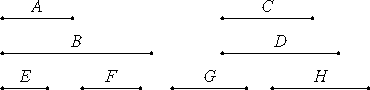# Proposition 27

Similar solid numbers have to one another the ratio which a cubic number has to a cubic number.

Let A and B be similar solid numbers.

I say that A has to B the ratio which cubic number has to cubic number.VIII.19

Since A and B are similar solid numbers, therefore two mean proportional numbers C and D fall between A and B.

Take E, F, G, and H, the least numbers of those which have the same ratio with A, C, D, and B, and equal with them in multitude.

VIII.2,Cor.

Therefore the extremes of them, E and H, are cubes. And E is to H as A is to B, therefore A also has to B the ratio which a cubic number has to a cubic number.

Therefore, similar solid numbers have to one another the ratio which a cubic number has to a cubic number.

Q.E.D.

## Guide

This proposition is analogous to the previous proposition about similar plane numbers.